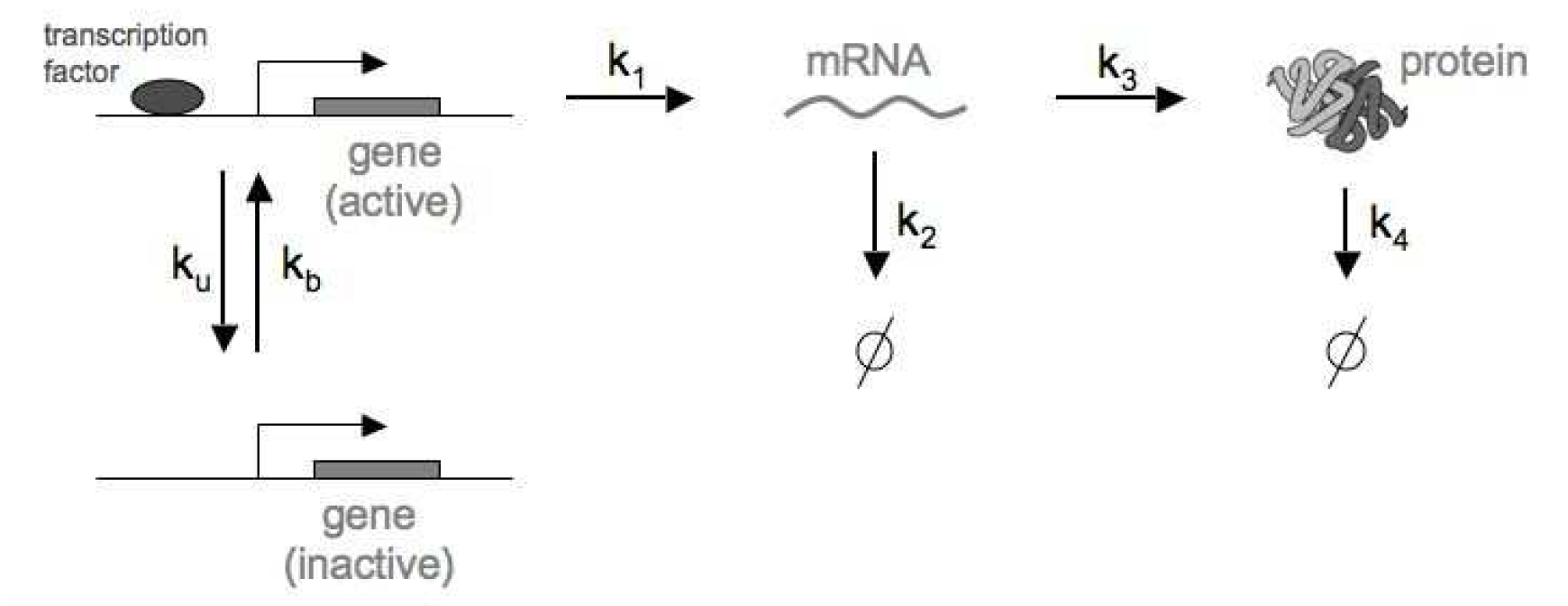# Molecular Population Dynamics as a Markov Process

## Stochastic simulation of transcriptional bursting

### Sampling fast from an exponential distribution

In the Gillespie algorithm, dwell times $$\tau$$ follow a exponential distribution.

The probability density function (PDF) is

$PDF(t) = W_R e^{-W_R\ t}.$

In order to sample a dwell time $$\tau$$, we used the cumulative probability function (CDF)

$CDF(\tau) = \int_0^{\tau} W_R\ e^{-W_R\ t}\ dt$

such that for a random number $$0\leq x\leq 1$$, the sampled dwell $$\tau$$ time is given by

$x = CDF(\tau).$

The CDF of the exponential distribution has a closed form given by

$CDF(\tau) = \int_0^{\tau} W_R\ e^{-W_R\ t} = 1 - e^{-W_R\ \tau}.$

Then, the sampled time is given by

$\tau = -\frac{1}{W_R}\ \log(1-x).$

If $$x$$ is a random number in $$[0,1]$$, then $$1-x$$ is also a random number in the same range, then we can take the sampled dwell time $$\tau$$ given by

$\tau = -\frac{1}{W_R}\ \log(x).$

### Implementing the Gillespie algorithm

For the stochastic process described in Figure 1, the reactions are given byFigure 1. Regulation of a single gene, transcription and translation, as well as RNA and Protein degradation processes.
r reaction propensity $$w_r$$ $$\eta(R)$$ $$\eta(P)$$
1 $$\mbox{Gene(I)} \overset{k_b}{\longrightarrow} \mbox{Gene(A)}$$ $$k_b$$ $$0$$ $$0$$
2 $$\mbox{Gene(A)} \overset{k_u}{\longrightarrow} \mbox{Gene(I)}$$ $$k_u$$ $$0$$ $$0$$
3 $$\mbox{Gene(A)} \overset{k_1}{\longrightarrow} RNA$$ $$k_1$$ $$+1$$ $$0$$
4 $$RNA \overset{k_2}{\longrightarrow} \emptyset$$ $$k_2 R$$ $$-1$$ $$0$$
5 $$RNA \overset{k_3}{\longrightarrow} Protein$$ $$k_3 R$$ $$0$$ $$+1$$
6 $$Protein \overset{k_4}{\longrightarrow} \emptyset$$ $$k_4 P$$ $$0$$ $$-1$$

Then the Gillespie algorithm works as follows

• Initialize at $$t = 0$$,

\begin{aligned} Gact &= 1,\\ R &= 0,\\ P &= 0.\\ \end{aligned}
• Keep a current count of $$t$$, $$Gact$$, $$R$$ and $$P$$.

• $$Gact = 1$$ if the gene is active (A) at time $$t$$, or
• $$Gact = 0$$ if the gene is inactive (I) at time $$t$$.
• $$R$$ is the number of RNA molecules at time $$t$$.
• $$P$$ is the number of protein molecules at time $$t$$.
• Sample a dwell time $$\tau$$ from a exponential distribution

$W_R\ e^{-W_R\ t},$

where the total propensity is given by

\begin{aligned} W_R = w_1 + w_4 + w_5 + w_6,\quad &\mbox{if}\, Gact = 0,\\ W_R = w_2 + w_3 + w_4 + w_5 + w_6,\quad &\mbox{if}\, Gact = 1.\\ \end {aligned}
• Update the current time $$t$$ as

$t\rightarrow t+\tau.$
• Sample one reaction $$r$$ according to their propensities

$P(r) = \frac{w_r}{W_R}.$
• Update $$Gact$$, $$R$$ and $$P$$

• If $$r = 1$$, then $$Gact=0 \rightarrow 1$$

• If $$r = 2$$, then $$Gact=1 \rightarrow 0$$

• If $$r = 3$$, then $$R \rightarrow R+1$$

• If $$r = 4$$, then $$R \rightarrow R-1$$

• If $$r = 5$$, then $$P \rightarrow P+1$$

• If $$r = 6$$, then $$P \rightarrow P-1$$

• Update the propensities according to the new values of $$Gact$$, $$R$$ and $$P$$.

• Go back to sampling a new dwell time, until a maximum time $$T$$.